CBSE Class 10 Sample Paper for 2022 Boards - Maths Standard [Term 2]

Class 10
Solutions of Sample Papers for Class 10 Boards

## ii) Find the total number of push-ups performed by Nitesh up to the day his goal is achieved.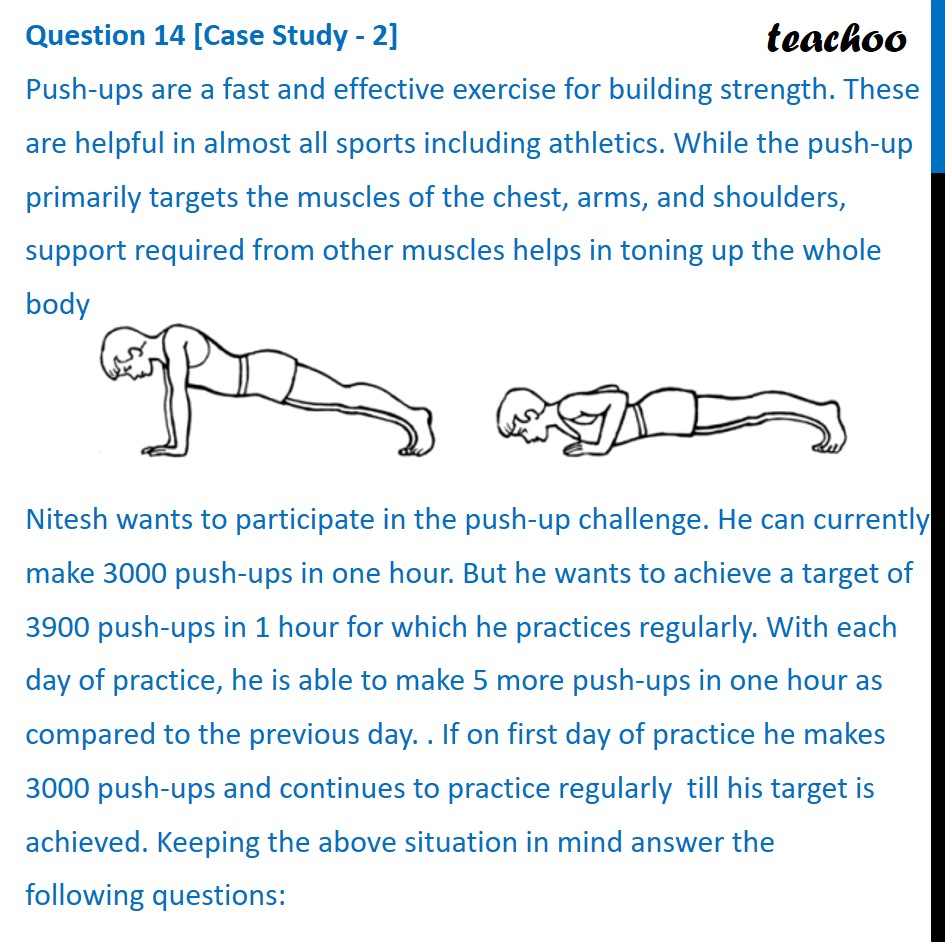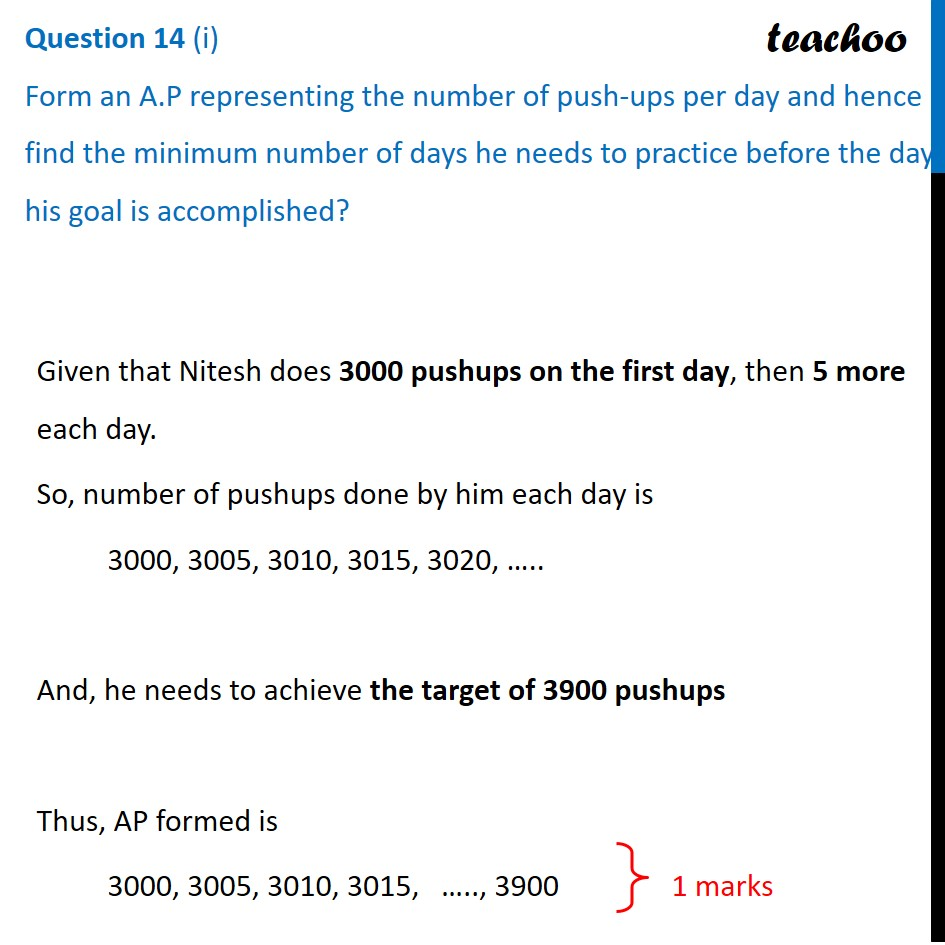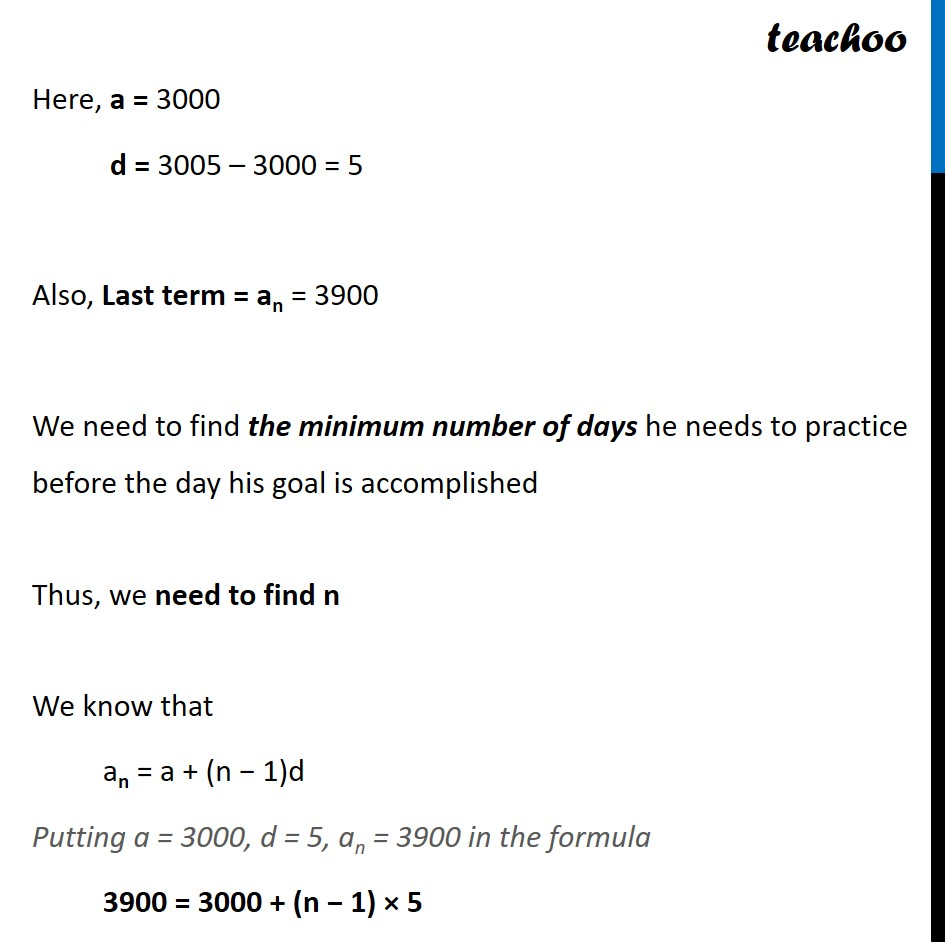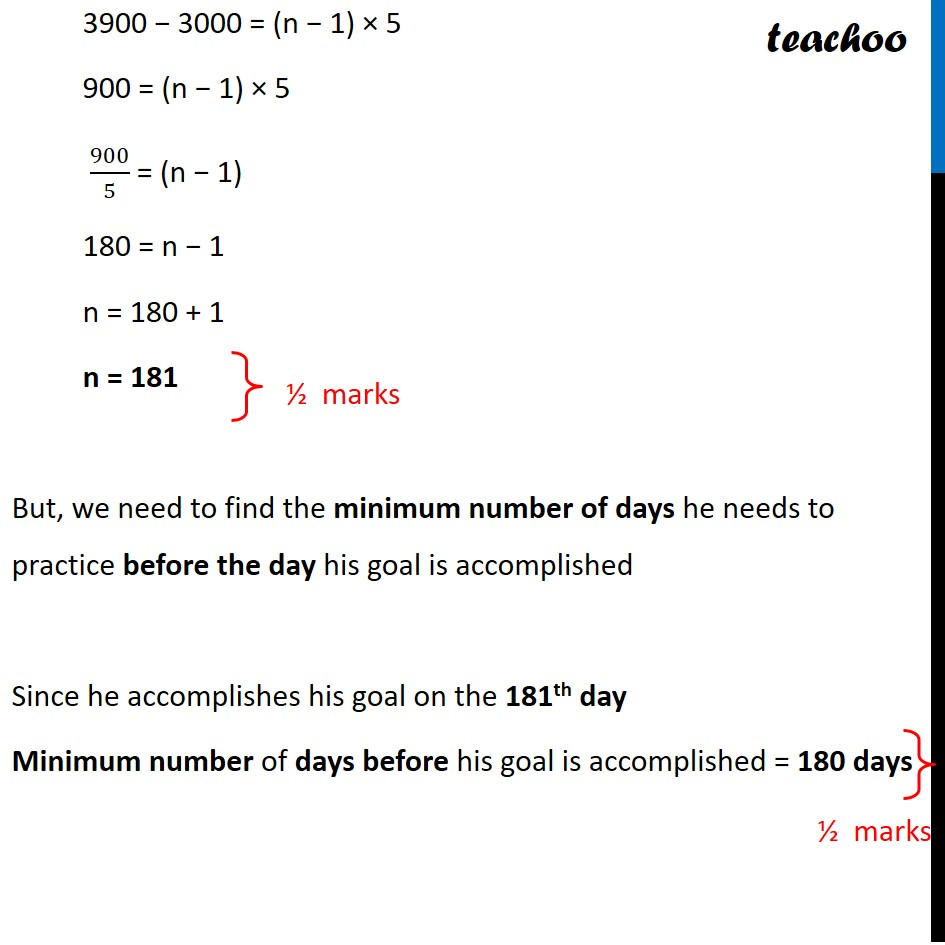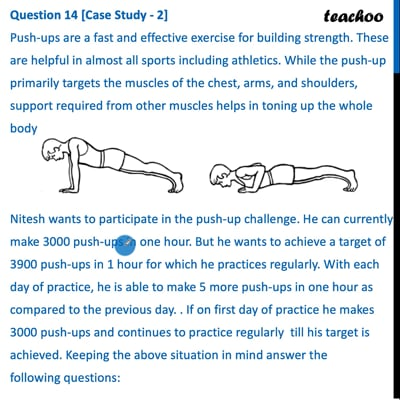This video is only available for Teachoo black users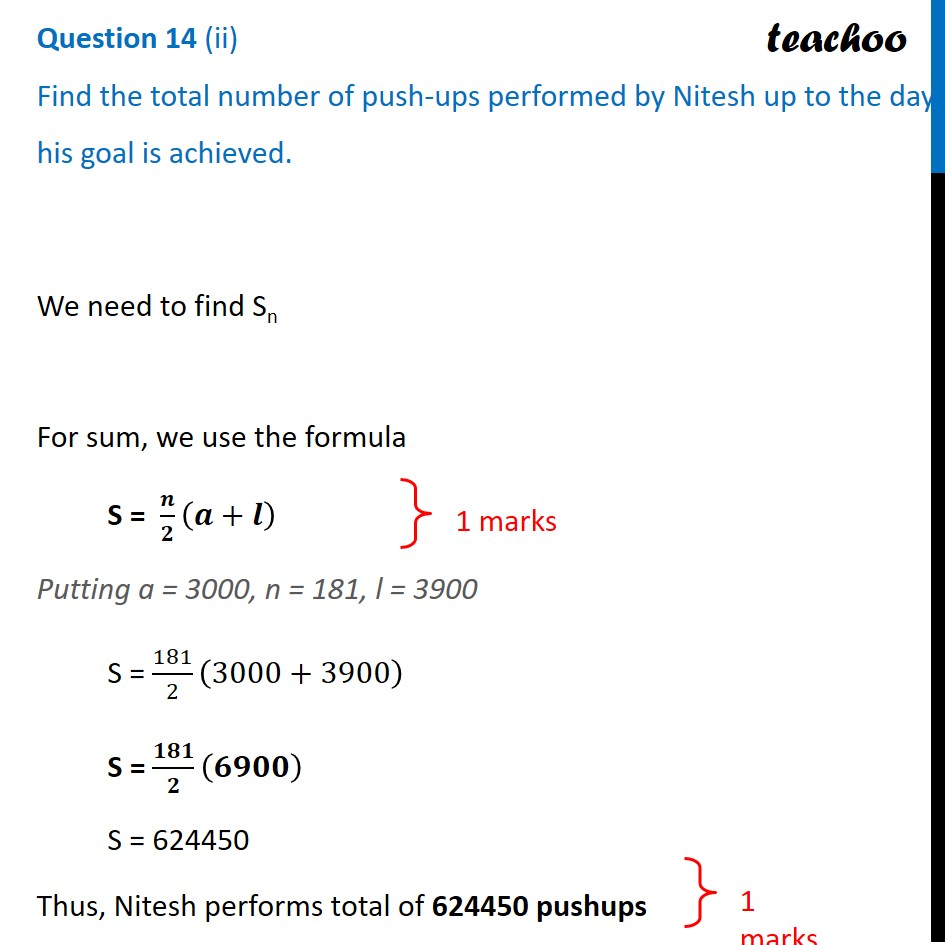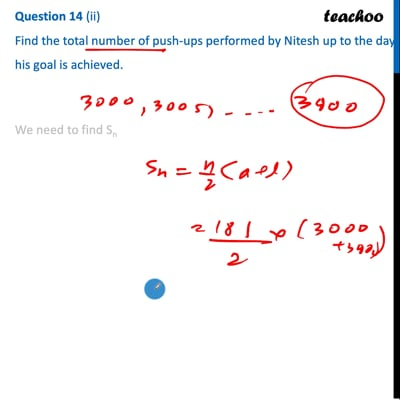This video is only available for Teachoo black users

### Transcript

Push-ups are a fast and effective exercise for building strength. These are helpful in almost all sports including athletics. While the push-up primarily targets the muscles of the chest, arms, and shoulders, support required from other muscles helps in toning up the whole body Nitesh wants to participate in the push-up challenge. He can currently make 3000 push-ups in one hour. But he wants to achieve a target of 3900 push-ups in 1 hour for which he practices regularly. With each day of practice, he is able to make 5 more push-ups in one hour as compared to the previous day. . If on first day of practice he makes 3000 push-ups and continues to practice regularly till his target is achieved. Keeping the above situation in mind answer the following questions: i) Form an A.P representing the number of push-ups per day and hence find the minimum number of days he needs to practice before the day his goal is accomplished? ii) Find the total number of push-ups performed by Nitesh up to the day his goal is achieved. Question 14 (i) Form an A.P representing the number of push-ups per day and hence find the minimum number of days he needs to practice before the day his goal is accomplished? Given that Nitesh does 3000 pushups on the first day, then 5 more each day. So, number of pushups done by him each day is 3000, 3005, 3010, 3015, 3020, ….. And, he needs to achieve the target of 3900 pushups Thus, AP formed is 3000, 3005, 3010, 3015, ….., 3900 Here, a = 3000 d = 3005 – 3000 = 5 Also, Last term = an = 3900 We need to find the minimum number of days he needs to practice before the day his goal is accomplished Thus, we need to find n We know that an = a + (n − 1)d Putting a = 3000, d = 5, an = 3900 in the formula 3900 = 3000 + (n − 1) × 5 3900 − 3000 = (n − 1) × 5 900 = (n − 1) × 5 900/5 = (n − 1) 180 = n − 1 n = 180 + 1 n = 181 But, we need to find the minimum number of days he needs to practice before the day his goal is accomplished Since he accomplishes his goal on the 181th day Minimum number of days before his goal is accomplished = 180 days Question 14 (ii) Find the total number of push-ups performed by Nitesh up to the day his goal is achieved. Question 14 (ii) Find the total number of push-ups performed by Nitesh up to the day his goal is achieved. We need to find Sn For sum, we use the formula S = 𝒏/𝟐 (𝒂+𝒍) Putting a = 3000, n = 181, l = 3900 S = 181/2 (3000+3900) S = 𝟏𝟖𝟏/𝟐 (𝟔𝟗𝟎𝟎) S = 624450 Thus, Nitesh performs total of 624450 pushups